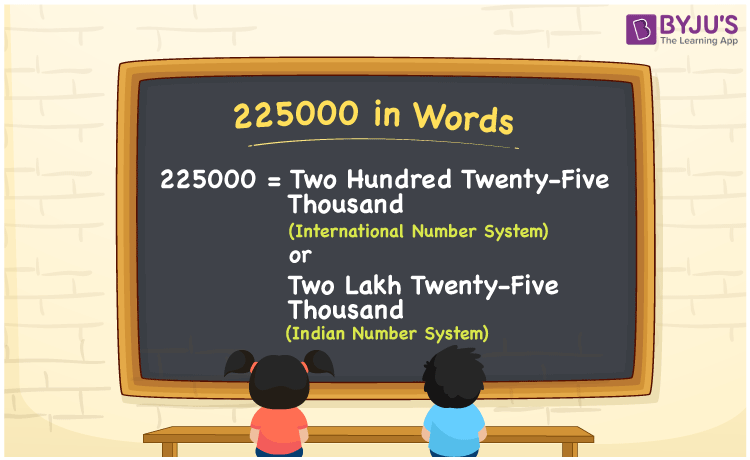# 225000 in Words

We can write 225000 in words as Two Hundred Twenty-five Thousand in the International number system and as Two Lakh Twenty-five Thousand  in the Indian number system. In this article, you will learn how to convert the cardinal number 225000 to words of both International and Indian number systems.

 225000 in words Two Hundred Twenty-five Thousand  (or) Two Lakh Twenty-five Thousand Two Hundred Twenty-five Thousand  (or) Two Lakh Twenty-five Thousand  in Numbers 225000

## 225000 in English Words

We generally write numbers in words using the English alphabet. Thus, we can read 225000 in English as “One thousand”.## How to Write 225000 in Words?

225000 in words – International number system

The below table depicts the place value chart for 225000 with respect to the International number system.

 Thousands Hundreds Tens Ones 225 0 0 0

Here, ones = 0, tens = 0, hundreds = 0, thousands = 250

Therefore, 225000 in words = 225 thousand = Two hundred Twenty-five thousand

225000 in words – Indian number system

Given below is the place value chart for 225000 in the Indian system.

 Lakhs Ten thousands Thousands Hundreds Tens Ones 2 2 5 0 0 0

Here, ones = 0, tens = 0, hundreds = 0, thousands = 5, ten thousands = 2, lakhs = 2

That means, 2 lakhs and 5 ten thousands

= 2 lakhs + (5 × 10) thousands

= 2 lakhs + 50 thousands

= Two Lakh Twenty-five Thousand

225000 is a natural number that precedes 225001 and succeeds 225499.

225000 in words – Two Hundred Twenty-five Thousand (or) Two Lakh Twenty-five Thousand

Is 225000 an odd number? – No

Is 225000 an even number? – Yes

Is 225000 a perfect square number? – No

Is 225000 a perfect cube number? – No

Is 225000 a prime number? – No

Is 225000 a composite number? – Yes

## Frequently Asked Questions on 225000 in Words

Q1

### How do you say 225000 in words?

We can say 225000 in words as Two Hundred Twenty-five Thousand (or) Two Lakh Twenty-five Thousand .
Q2

### How do you write Rs. 225000 in words for a cheque?

For a cheque, we write Rs. 225000 in words as “Two Lakh Twenty-five Thousand rupees only”.
Q3

### Write the answer in words for 135000 + 115000.

135000 + 115000 = 225000
Therefore, the value of 135000 + 115000 in words is Two Hundred Twenty-five Thousand (or) Two Lakh Twenty-five Thousand.